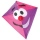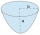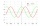# Square (second power, quadratic) + expression of a variable from the formula - math problems

#### Number of problems found: 46

• Cube surfce2volumeCalculate the volume of the cube if its surface is 150 cm2.
• 1 page1 page is torn from the book. The sum of the page numbers of all the remaining pages is 15,000. What numbers did the pages have on the page that was torn from the book?
• The cubeThe cube has a surface area of 216 dm2. Calculate: a) the content of one wall, b) edge length, c) cube volume.
• Triangular prismThe triangular prism has a base in the shape of a right triangle, the legs of which is 9 cm and 40 cm long. The height of the prism is 20 cm. What is its volume cm3? And the surface cm2?
• Diameter = heightThe surface of the cylinder, the height of which is equal to the diameter of the base, is 4239 cm square. Calculate the cylinder volume.
• Trip with compassDuring the trip, Peter went 5 km straight north from the cottage, then 12 km west and finally returned straight to the cottage. How many kilometers did Peter cover during the whole trip?
• A kiteChildren have a kite on an 80m long rope, which floats above a place 25m from the place where children stand. How high is the dragon floating above the terrain?
• The ballThe ball has a radius of 2m. What percentage of the surface and volume is another sphere whose radius is 20% larger?
• Area to volumeIf the surface area of a cube is 486, find its volume.
• Uboid volumeCalculate the cuboid volume if the walls are 30cm², 35cm², 42cm²
• The parabolic segmentThe parabolic segment has a base a = 4 cm and a height v = 6 cm. Calculate the volume of the body that results from the rotation of this segment a) around its base b) around its axis.
• Triangular pyramidA regular tetrahedron is a triangular pyramid whose base and walls are identical equilateral triangles. Calculate the height of this body if the edge length is a = 8 cm
• The schoolyardThe schoolyard had the shape of a square with an 11m side. The yard has been enlarged by 75 m2 and has a square shape again. How many meters was each side of the yard enlarged?
• Quarter circleWhat is the radius of a circle inscribed in the quarter circle with a radius of 100 cm?
• Surface area of the topA cylinder is three times as high as it is wide. The length of the cylinder’s diagonal is 20 cm. Find the surface area of the top of the cylinder.
• Substitution methodSolve goniometric equation: sin4 θ - 1/cos2 θ=cos2 θ - 2
• Sum of squaresThe sum of squares above the sides of the rectangular triangle is 900 cm2. Calculate content of square over the triangle's hypotenuse.
• Cube surface and volumeThe surface of the cube is 500 cm2, how much cm3 will be its volume?
• Two diagonalsThe rhombus has a side length 12 cm and length of one diagonal 21 cm. What is the length of the second diagonal?
• Cube surface and volumeFind the surface of the cube with a volume of 27 dm3.

Do you have an interesting mathematical word problem that you can't solve it? Submit a math problem, and we can try to solve it.

We will send a solution to your e-mail address. Solved examples are also published here. Please enter the e-mail correctly and check whether you don't have a full mailbox.

Please do not submit problems from current active competitions such as Mathematical Olympiad, correspondence seminars etc...